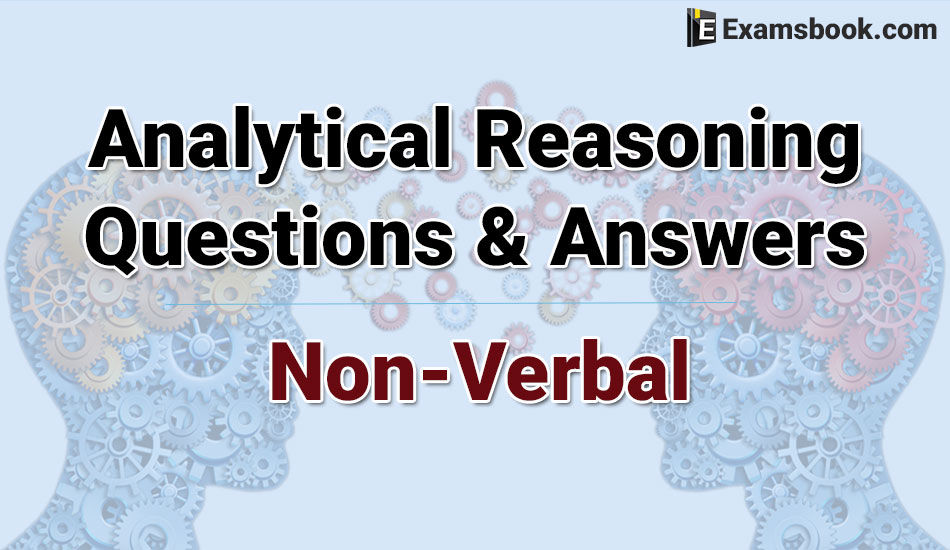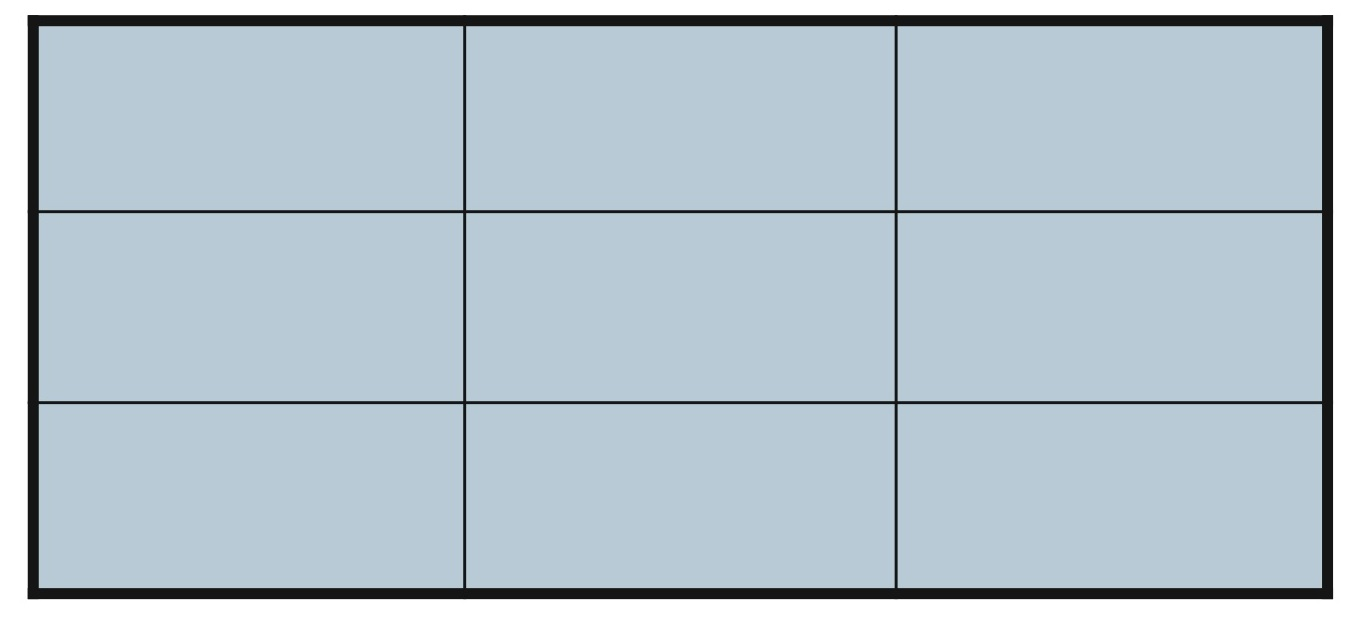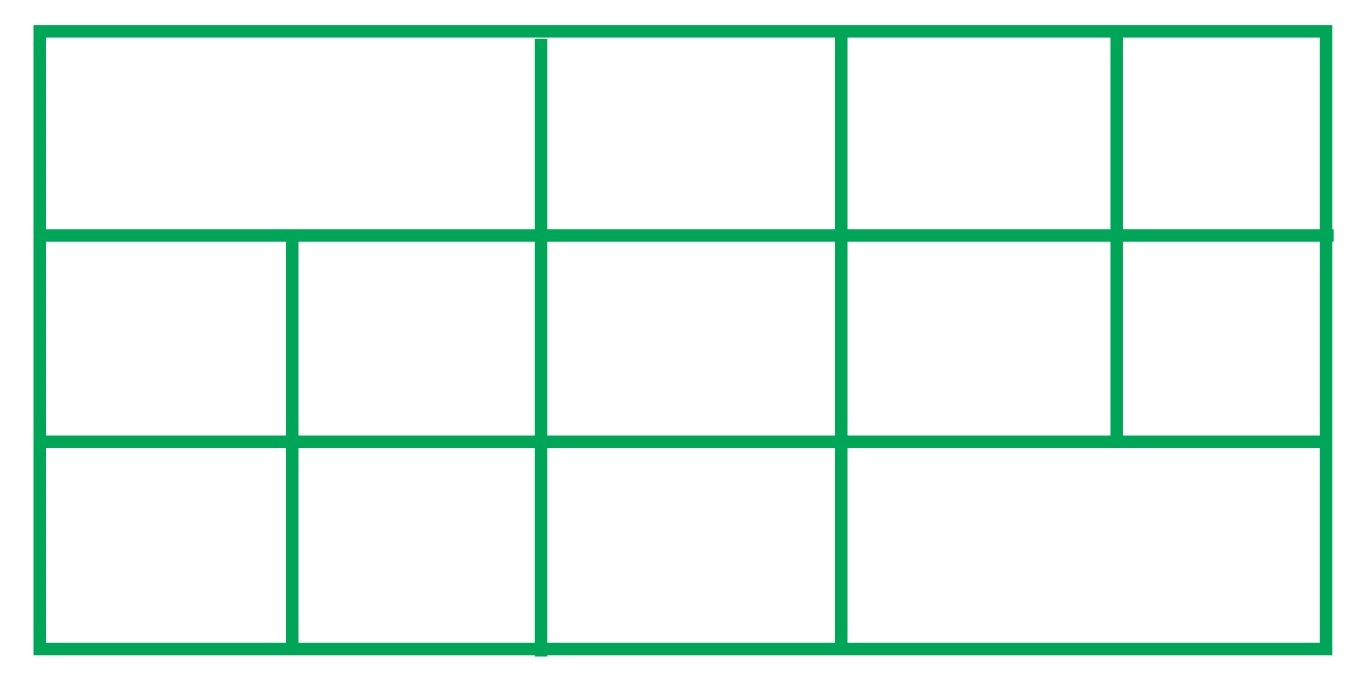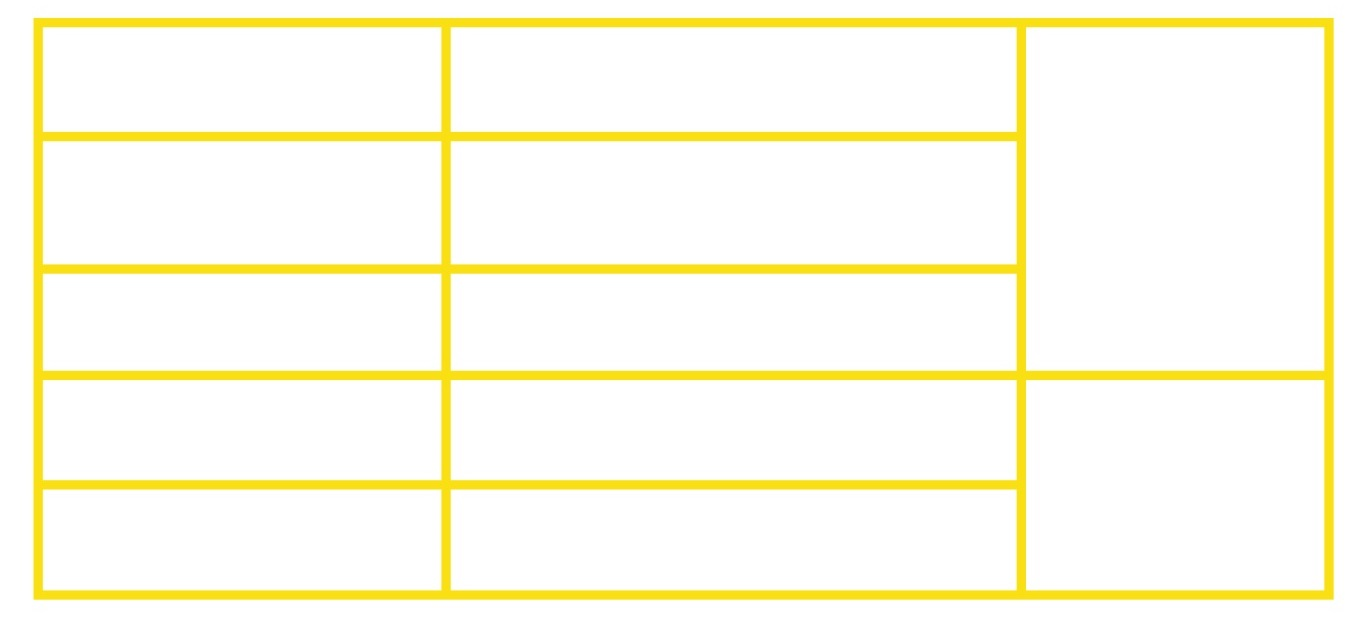• Save

Analytical Reasoning Questions and Answers - Non Verbal Reasoning

4 months ago 4.6K ViewsMost of the students confused in finding analytical reasoning questions and answers of Non-Verbal Reasoning that where they can practice best. They want to prepare important analytical reasoning questions and answers of Non-Verbal Reasoning without wasting their time.

So, here you can get the important and selective analytical reasoning questions and answers for SBI, SSC, Bank exam and other competitive exams. Let's do some practice work.

Analytical Reasoning Questions and Answers Non-Verbal problems for competitive exams:

Q.1. Find the number of rectangles?(A) 19

(B) 28

(C) 27

(D) 36

Ans .   D

Q.2. Find the number of square?(A) 10

(B) 18

(C) 15

(D) 19

Ans .   A

Q.3. Find the number of triangles?(A) 16

(B) 13

(C) 9

(D) 7

Ans .   A

Q.4. Find the number of triangles?(A) 12

(B) 14

(C) 15

(D) 13

Ans .   C

Q.5. Find the number of square?(A) 13

(B) 14

(C) 15

(D) 16

Ans .   D

Q.6. Find the number of square?(A) 10

(B) 16

(C) 19

(D) 12

Ans .   C

Q.7.Find the number of rectangles?(A) 24

(B) 27

(C) 30

(D) 28

Ans .   B

Q.8. Find the number of square?(A) 6

(B) 9

(C) 7

(D) 10

Ans .   B

Students can comment or ask me anything in comment sections regarding analytical reasoning questions and answers of Non-Verbal Reasoning. To more practice, analytical reasoning questions and answers - (Non-Verbal Reasoning) visit the next page.